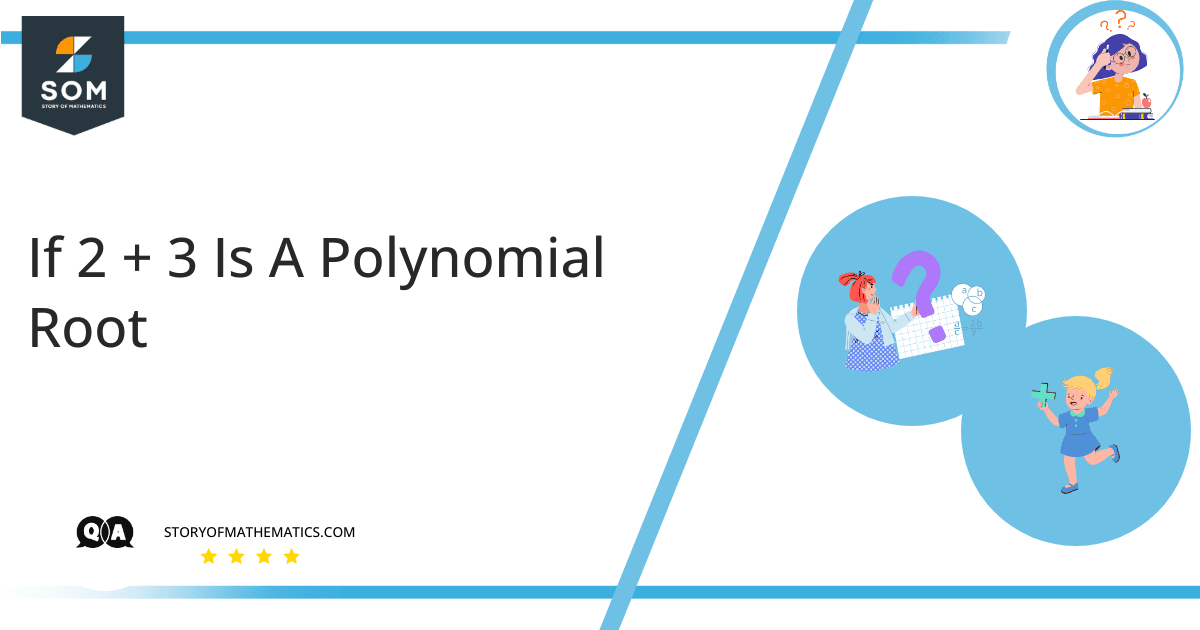# If 2 + sqrt(3) is a polynomial root, name another root of the polynomial, and explain how you know it must also be a root.The aim of this question is to qualitatively evaluate the roots of a polynomial using prior knowledge of algebra.

As an example, let’s consider a standard quadratic equation:

$a x^{ 2 } \ + \ b x \ + \ c \ = \ 0$

The roots of such a quadric equation are given by:

$\lambda_{1,2} \ = \ \dfrac{ -b \ \pm \ \sqrt{ b^{ 2 } \ – \ 4 a c } }{ 2 a }$

Here, one may notice that the two roots are conjugates of each other.

A conjugate pair of roots is the one where two roots have the same non-square root term but their square root terms are equal and opposite in sign.

Given that:

$\lambda_1 \ = \ 2 \ + \ \sqrt{ 3 }$

If we assume that the polynomial has a degree of 2:

$a x^{ 2 } \ + \ b x \ + \ c \ = \ 0$

Then we know that the roots of such a quadric equation are given by:

$\lambda_{1,2} \ = \ \dfrac{ -b \ \pm \ \sqrt{ b^{ 2 } \ – \ 4 a c } }{ 2 a }$

This shows that the two roots $\lambda_1$ and $\lambda_2$ are conjugates of each other. So if $2 \ + \ \sqrt{ 3 }$ is one root then $2 \ – \ \sqrt{ 3 }$ must be the other root.

Here, we have assumed that the equation is quadratic. However, this fact is true for any polynomial of order higher than two.

## Numerical Result

If $2 \ + \ \sqrt{ 3 }$ is one root, then $2 \ – \ \sqrt{ 3 }$ must be the other root.

## Example

Given the equation $x^{ 2 } \ + \ 2 x \ + \ 4 \ = \ 0$, find its roots.

Comparing the given equation with the following standard quadratic equation:

$a x^{ 2 } \ + \ b x \ + \ c \ = \ 0$

We can see that:

$a \ = \ 1, \ b \ = \ 2 \text{ and } \ c \ = \ 4$

Roots of such a quadric equation are given by:

$\lambda_{1,2} \ = \ \dfrac{ -b \ \pm \ \sqrt{ b^{ 2 } \ – \ 4 a c } }{ 2 a }$

Substituting values:

$\lambda_{1,2} \ = \ \dfrac{ -2 \ \pm \ \sqrt{ 2^{ 2 } \ – \ 4 ( 1 ) ( 4 ) } }{ 2 ( 1 ) }$

$\lambda_{1,2} \ = \ \dfrac{ -2 \ \pm \ \sqrt{ 4 \ – \ 16 } }{ 2 }$

$\lambda_{1,2} \ = \ \dfrac{ -2 \ \pm \ \sqrt{ -12 } }{ 2 }$

$\lambda_{1,2} \ = \ -1 \ \pm \ \sqrt{ -3 }$

$\lambda_{1,2} \ = \ -1 \ \pm \ \sqrt{ 3 } i$

Which are the roots of the given equation.